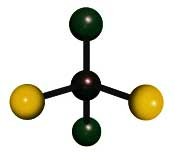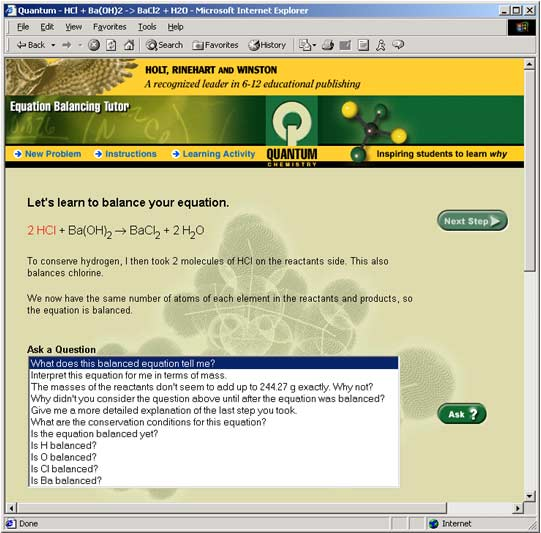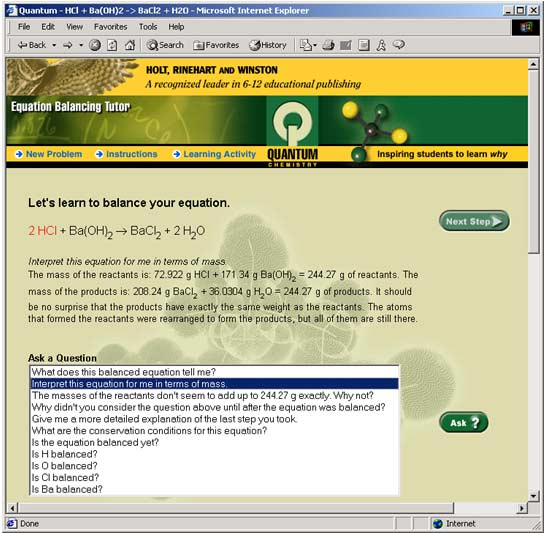This easy to use exercise displays the key functions of the Equation Balancing Tutor. Once this exercise is completed, students will be familiar and comfortable with the Tutor, giving them maximum benefit as they progress with their chemistry studies.

Similar to a human tutor, the Quantum Tutor deliberately offers a variety of wordings and numbers in its examples and explanations every time you use it. The ideas and concepts are the same. So, be prepared — you may not see the exact same words or numbers that appear in these sample screens. Know that you are learning the concepts and the why behind the answers, not just memorizing facts and procedures.

Keep in mind that the best opportunity to learn occurs when mistakes are made — and that is when the full power of the Quantum Tutor is realized.

Teachers may choose to print out this document and use it as an assignment in the classroom or for homework. Space has been provided for students to write in their responses.

# Learning Objectives:

• Students will be able to balance equations using the Tutor, either by choosing an equation from a menu or entering their own original equation.# Overview of Exercise:

Steps 1 through 6:  Students enter an equation, balance it, and ask the Tutor various questions along the way.

Step 7:  Students pick an equation to be balanced from the Tutor's menu, balance it, and use the Questions Menu to analyze the problem.

# Step 1: Getting Started

To use this Activity, you will need to toggle back and forth between this page and the Equation Balancing Tutor. You will have multiple windows open, and some windows may be hidden from view. If you are using a PC, look at the Windows toolbar to see which windows are running. Find the title of the window that you want, and click on the title to make that window the active window. If you are using a Mac, you may need to minimize all of the browser windows to find the one that you want. At any time you may click Instructions at the upper left of your screen for information about the operation of the Tutor. The main window will look like this:

### Figure 1: Equation Balancing Tutor main window.

There are two ways for the Tutor to help you balance an equation. You can either enter your own equation into the top box labeled Enter an Equation, or you can pick an equation from the Choose an Equation menu below it. Either way, the Tutor will show you how to balance the equation. Start by entering a sample equation (see Step 2).

# Step 2:  Enter an equation

The Tutor allows us to enter the equations unformatted, or as plain text. In the box Enter an Equation enter the following equation, as shown:

HCl + Ba(OH)2 -> BaCl2 + H2O

### Figure 2: Entering an equation.

The equation you just entered is the reaction of hydrochloric acid with barium hydroxide. To begin, Click on the green OK button on the right. You will see a window that looks like this:

Notice that you don't need to type in subscripted numbers because the Tutor properly displays them for you.

# Step 3: Take the first step in balancing the equation

The Tutor will balance the equation step by step. Click on the green Next Step button at the right. The result will look like this:

### Figure 4: The Tutor takes the first step.

The Tutor highlights the change it made to the equation and gives you a short explanation.

The Tutor can do more than simply balance equations. It can also help you answer questions you might have. Let's ask some questions of the Tutor.

a)   In the first step, the oxygen (O) atoms were balanced first. Let's have the Tutor explain this step in more detail.

Click on the question, "Give me a more detailed explanation of the last step you took." to highlight it. Then, click on the green Ask? button to see the Tutor's answer.

### Figure 5: The Tutor answers the question:  "Give me a more detailed explanation of the last step you took."

b)   Now click the question "Is the equation balanced yet?" then click Ask? Then, ask "Is H balanced?" to get an explanation of why hydrogen is not yet balanced. Check to be sure the oxygens (O) are balanced by asking "Is O balanced?" The Tutor will confirm that oxygen (O) is balanced. But there is still work to do.

# Step 5:  Take another step

To finish balancing the equation, click the green Next Step button and see what happens.### Figure 6: The Tutor takes a second step and balances the entire equation.

The second step results in the final balanced equation. What question could you ask the Tutor to show that the equation is balanced?

Which element did the Tutor balance next to bring all the other elements into balance?  (Hint:  Look at the compound highlighted in red to help you.)

Why didn't the Tutor balance barium (Ba) or chlorine (Cl) after oxygen (O)?

# Step 6:  Ask some more questions

Now that the equation is balanced, ask the Tutor a few questions about the balanced equation. Click on the following two questions and see what the Tutor has to say about each:

"Interpret this equation for me in terms of mass."

"What does this balanced equation tell me?"### Figure 7: The Tutor answers the question:  "Interpret this equation for me in terms of mass".

Summarize what you learned from both questions in the space provided.

Now that you are finished, scroll down to the bottom of the screen. There, you will see a record of every step you took and every question you asked of the Tutor.

### Figure 8: The Tutor always displays the transcript.

This record is a "transcript" of the work you did so far. It is always there if you need to review a question or step when you use the Tutor. Because of the valuable information contained in this transcript, you will find that reviewing it will be very worthwhile. In addition to the correct answers and how to arrive at them, the Tutor also offers excellent suggestions on useful ways to think about the concepts.

# Step 7: Start a new problem from the menu of equations

Remember that the Tutor also allows you to pick an equation instead of entering your own. Let's try that now. Click on the New Problem button, located in the upper left corner of the screen.

Highlight the following equation from the menu, as shown below, and click the OK button.

SO2 + O2 -> SO3

### Figure 9: Main menu to begin a new problem.

Click on the Next Step button to take the first step. Then, you will see the following:

### Figure 10: The Tutor takes the first step.

Continue balancing the equation until you are finished. Along the way, use the questions menu to answer the following questions:

Why did the Tutor multiply through by 2 on the first step?

Which element did the Tutor balance first?

Which element did the Tutor balance last?

What question did you ask the Tutor to make sure the final equation was balanced?

The two problems you have just completed illustrate many of the major features of the Quantum Tutors. The program has tutored you through the entire process by encouraging you to bring together all related background information and concepts. You have reviewed oxidation numbers, ionic charges, chemical symbols, formula writing, naming compounds, and conservation of atoms.

Your challenge is to continue to bring this knowledge together for each new example. With these skills and concepts now clearly associated in your thinking you are much better equipped to handle the next equation and, of course, the Tutor stands ready to assist you every step of the way.As you move onto other problems, begin to predict what the Tutor will do next and what explanation it will provide for each new step. When you reach a point where your predictions and explanations begin to match those of the Tutor, then you will have mastered this concept.